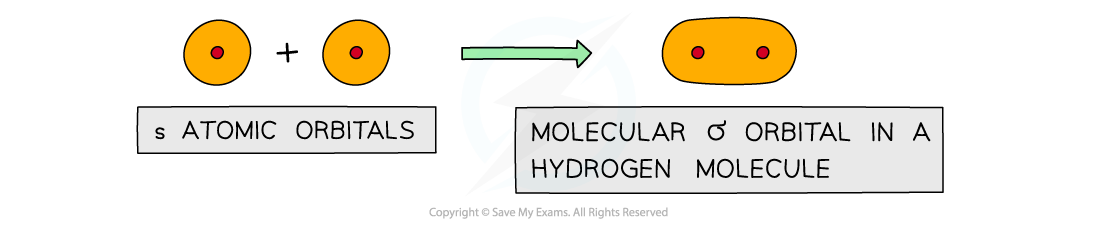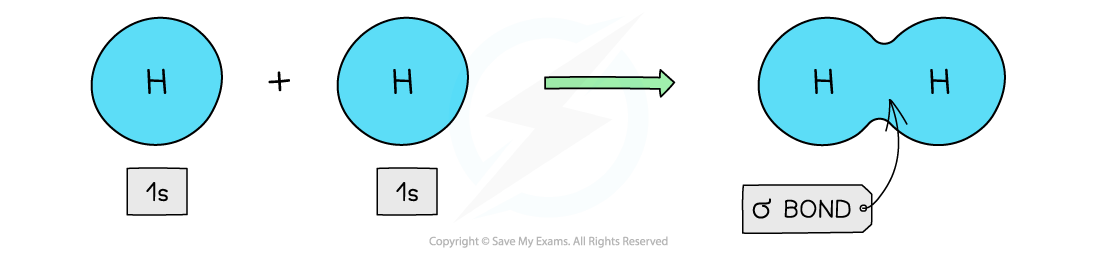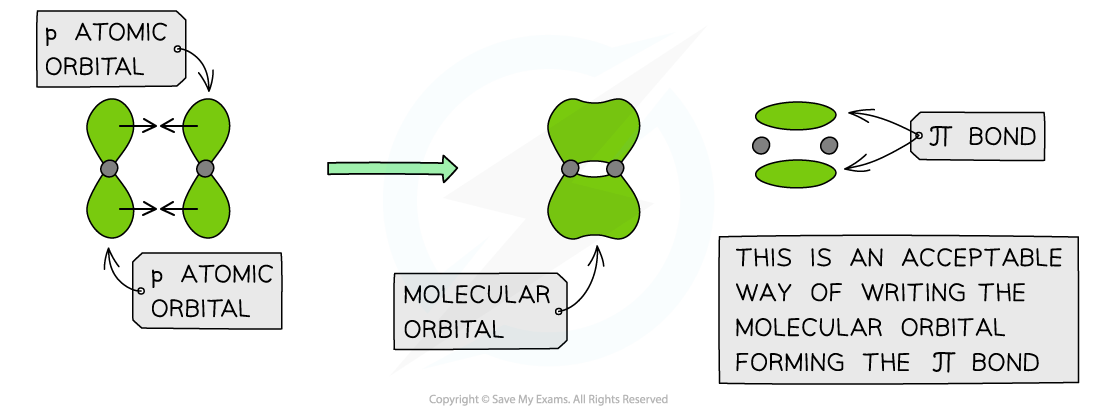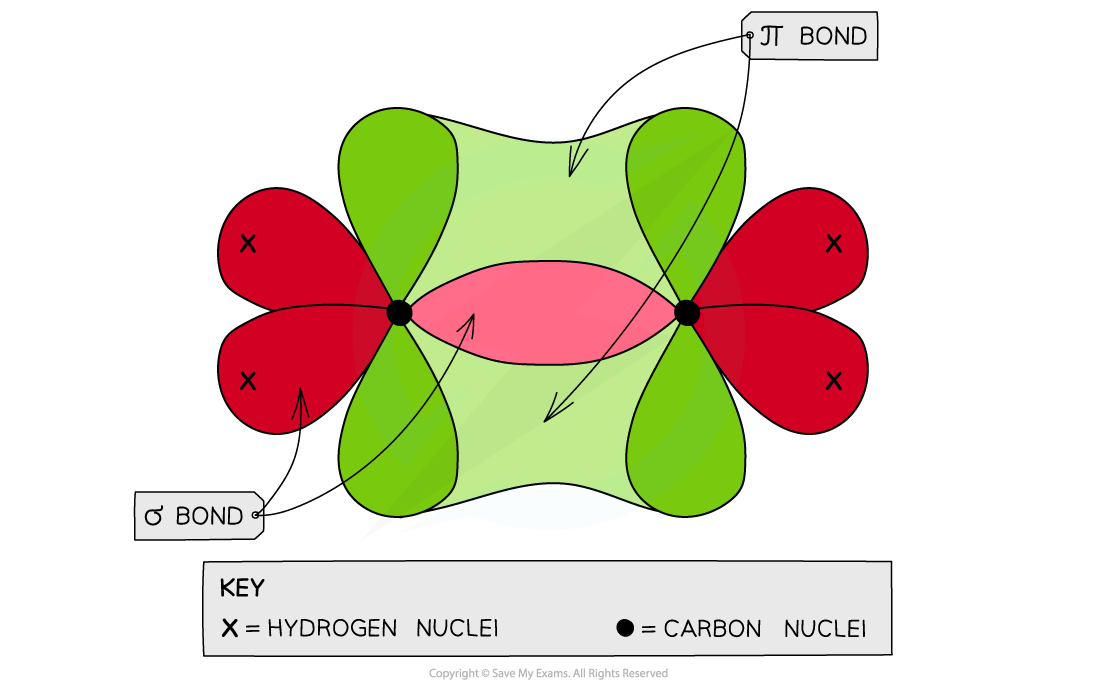# Edexcel A Level Chemistry:复习笔记3.3.2 Bonding in Alkenes

### Bonding in Alkenes

• Each carbon atom has four electrons in its outer shell (electronic configuration: 1s22s22p2)
• Carbon atoms share these four electrons in four covalent bonds with other atoms to achieve a full outer shell configuration
• These electrons are found in orbitals within the respective atoms
• When forming a covalent bond, the orbitals overlap in such a way to form two types of bonds
• Sigma bonds (σ)
• Pi bonds (π)
• When carbon atoms use only three of their electron pairs to form a σ bond, each carbon atom will have a p orbital which contains one spare electron
• When the p orbitals of two carbon atoms overlap with each other, a π bond is formed (the π bond contains two electrons)
• The two orbitals that form the π bond lie above and below the plane of the two carbon atoms to maximise bond overlap

#### σ bonds

• Sigma (σ) bonds are formed from the end to end overlap of atomic orbitals
• s orbitals overlap this way as well as p orbitalsSigma orbitals can be formed from the end to end overlap of s orbitals

• The electron density in a σ bond is symmetrical about a line joining the nuclei of the atoms forming the bond
• The pair of electrons is found between the nuclei of the two atoms
• The electrostatic attraction between the electrons and nuclei bonds the atoms to each other

#### Hydrogen

• The hydrogen atom has only one s orbital
• The s orbitals of the two hydrogen atoms will overlap to form a σ bond#### π bonds

• Pi (π) bonds are formed from the sideways overlap of adjacent p orbitals
• The two lobes that make up the π bond lie above and below the plane of the σ bond
• This maximises overlap of the p orbitals
• A single π bond is drawn as two electron clouds, one arising from each lobe of the p orbitals
• The two clouds of electrons in a π bond represent one bond containing two electronsπ orbitals can be formed from the sideways overlap of p orbitals

#### Ethene

• Each carbon atom uses three of its four electrons to form σ bonds
• Two σ bonds are formed with the hydrogen atoms
• One σ bond is formed with the other carbon atom
• The fourth electron from each carbon atom occupies a p orbital which overlaps sideways with another p orbital on the other carbon atom to form a π bond
• This means that the C-C is a double bond: one σ and one π bondEach carbon atom in ethene forms two sigma bonds with hydrogen atoms and one σ bond with another carbon atom. The fourth electron is used to form a π bond between the two carbon atoms